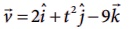Home | Kinematics: Multiple choice questions with answers

# Kinematics: Multiple choice questions with answers

Multiple choice questions with Answers, Solution and Explanation - Physics : Kinematics

Kinematics

1. Which one of the following Cartesian coordinate systems is not followed in physics?Ans: d

2. Identify the unit vector in the following.Ans: d

3. Which one of the following physical quantities cannot be represented by a scalar?

(a) Mass

(b) length

(c) momentum

(d) magnitude of acceleration

4. Two objects of masses m1 and m2 fall from the heights h1 and h2 respectively. The ratio of the magnitude of their momenta when they hit the ground isAns: c

5. If a particle has negative velocity and negative acceleration, its speed

(a) increases

(b) decreases

(c) remains same

(d) zero

6. If the velocity is, then the magnitude of acceleration at t = 0.5 s is

(a) 1m s-2

(b) 2 m s-2

(c) zero

(d) -1 m s-2

7. If an object is dropped from the top of a building and it reaches the ground at t = 4 s , then the height of the building is (ignoring air resistance) (g = 9.8 ms–2)

(a) 77.3 m

(b) 78.4 m

(c) 80.5 m

(d) 79.2 m

8. A ball is projected vertically upwards with a velocity v. It comes back to ground in time t. Which v-t graph shows the motion correctly?Ans: c

9. If one object is dropped vertically downward and another object is thrown horizontally from the same height, then the ratio of vertical distance covered by both objects at any instant t is

(a) 1

(b) 2

(c) 4

(d) 0.5

10. A ball is dropped from some height towards the ground. Which one of the following represents the correct motion of the ball?Ans: a

11. If a particle executes uniform circular motion in the xy plane in clock wise direction, then the angular velocity is in

(a) +y direction

(b) +z direction

(c) -z direction

(d) -x direction

12. If a particle executes uniform circular motion, choose the correct statement

(a) The velocity and speed are constant.

(b) The acceleration and speed are constant.

(c) The velocity and acceleration are constant.

(d) The speed and magnitude of acceleration are constant.

13. If an object is thrown vertically up with the initial speed u from the ground, then the time taken by the object to return back to ground is

(a) u2/2g

(b) u2/g

(c) u/2g

(d) 2u/g

14. Two objects are projected at angles 30° and 60° respectively with respect to the horizontal direction. The range of two objects are denoted as R30̊ and R60̊. Choose the correct relation from the following

(a) R30̊ = R60̊

(b) R30̊  =4R60̊

(c)R30̊  = R60̊ /2

(d) R30̊  =2 R60̊

15. An object is dropped in an unknown planet from height 50 m, it reaches the ground in 2 s . The acceleration due to gravity in this unknown planet is

(a) g = 20 m s-2

(b) g = 25 m s-2

(c) g = 15 m s-2

(d) g = 30 m s-2

1) d 2) d 3) c 4) c 5) a

6) a 7) b 8) c 9) a 10) a

11) c 12) d 13) d 14) a 15) b

Tags : Physics , 11th Physics : UNIT 2 : Kinematics
Study Material, Lecturing Notes, Assignment, Reference, Wiki description explanation, brief detail
11th Physics : UNIT 2 : Kinematics : Kinematics: Multiple choice questions with answers | Physics